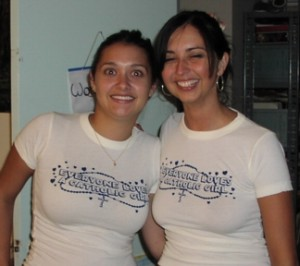# Canonical correlation: What I was thinking about today

August 30, 2010 | 1 Comment

I probably hadn’t thought about canonical correlation in twenty years, but then a problem came up this week where it was the exact technique I needed. What made me laugh, though, is the particular problem I was dealing with twenty years ago had school achievement measures – tests of English, Mathematics, Science and Social Studies – as the dependent variables and the problem I was dealing with this week had, you guessed it, school achievement measures as the dependent variables.

So, I thought I’d ramble on about canonical correlation for a while…Canonical correlation is used when you want to maximize the correlation between a set of X variables and a set of Y variables. For example, you might want to know how much teachers can affect student performance. You have a set of teacher factors; years of experience, percentage of time spent in hands-on activities, percentage of time spent on classroom discipline, minutes per week spent on preparation, minutes per week spent on grading. You have a set of student outcome variables, math achievement, reading achievement and science achievement.

You could do three multiple regression equations and maximize the explained variance in each dependent variable individually. However, hypothetically speaking, what if you found that increasing classroom structure increased achievement in science but decreased it in math? If you’re an elementary teacher, it’s probably hard to relax and increase the classroom rules during the day depending on the subject. School achievement is one of the few good candidates that leaps to mind for canonical correlation because you have multiple dependent variables and it is hard to argue one is more important than the other. We want kids who can read AND do math.

In a simple linear regression, we are calculating the covariance between two variables, X and Y. (The standardized form of the covariance is correlation.)

In a regular multiple regression equation we are trying to select the set of regression coefficients that maximize the covariance between a set of X variables and a single variable. To get a multiple correlation, we apply those regression coefficients to the X variables for each individual. We get a predicted score for that individual, the Y-hat. The correlation between the predicted Y and the actual Y is the multiple correlation.

In a canonical correlation, we go one step further. We have a set of X variables and we are trying to maximize the covariance between TWO matrices. (If you remember your normal equations from college, with multiple regression you had an X matrix and a Y vector – a vector, in statistical terms, being just a column of numbers, not to be confused with the geometric term of the same name, just like one should not confuse a three-way interaction in ANOVA by the event of the same name in pornography, of which I hear a great deal more of the latter than the former can by found on the Internet. Extremely odd when you consider that the initial motivation for development of the Internet was assistance of scientific research and not distribution of pornography. It’s true. You can look it up.)

ANYWAY … multiple regression maximizes the covariance between the X matrix and the Y vector while canonical correlation maximizes the covariance between the X matrix and the Y matrix.

I was going to say more about this but I have to finish my second WUSS paper on procedures. Speaking of SAS, if you wanted to do a canonical correlation with SAS, it’s very easy. You simply type:

```proc cancorr data = datasetname ; var first-list-of-variable names ; with second-list-of-variablenames ;```

Of course, there are a ton of options. One point really worth making is that you can analyze the covariance matrix, correlation matrix and other types of matrices. This is useful because listwise deletion is a common problem in analyses with a large number of variables, that is, if a person is missing just one out of ten variables he or she is dropped from the analysis. So, if you have ten variables each of which are only missing 4% of the data you can easily end up with 20-40% of your subjects dropped from the analysis. (It would be a little odd if it was 40%, but that’s another topic.)

Speaking of SPSS, even though we weren’t, although there are usually pointy-clicky things for just about every statistical procedure in SPSS I could not find it for canonical correlation. No big deal, just open a syntax window and use a MANOVA statement, like so (this uses the example from the anorexic data set included in the SPSS samples).

MANOVA weight mens fast with binge vomit purge
/discrim all alpha(1)
/Print = sig(eigen dim) .

I would like to say a lot more about this but I promised to have a paper on procedures novice programmers need to learn and I am kind of guessing that the conference organizers would give me “THAT LOOK” if I suggested that CANCORR was one of those procedures. I know the exact look. It is the one Maria gave Dennis when he asked her if she had thought of re-setting the programmable random access memory on her computer when she had a problem. She said,

“No, but I thought of drop-kicking it.”Name (required)

Email (required)

Website